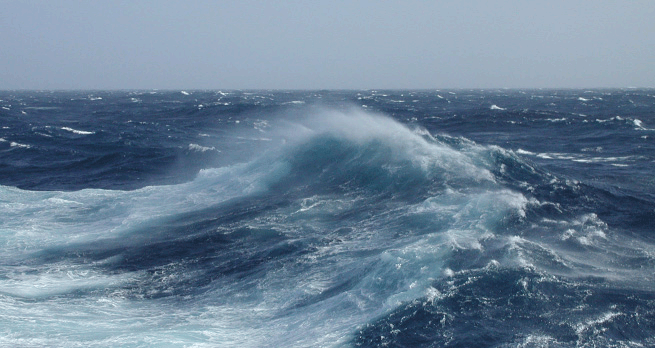Science, Maths & Technology

### Become an OU studentThe oceans

Start this free course now. Just create an account and sign in. Enrol and complete the course for a free statement of participation or digital badge if available.

# 3.4 The vertical distribution of properties

The properties of the ocean surface are changed by (E-P) and solar input but, as you have already seen in Figure 7, there are variations in these properties with depth.

• How can surface changes be carried deeper into the ocean?

• The energy can be transported downwards by the conduction of heat and diffusion of salt, and by being mixed up by the wind.

Both conduction and diffusion in the ocean are slow processes that can effectively be ignored. It is the effect of the wind on the surface of the ocean that dominates. Wind creates turbulence, thus mixing water to create a layer of almost constant temperature, salinity and density called the wind-mixed layer (Figure 11).Figure 11 An expanded plot of the upper 200 m of Figure 7.

Figure 11a shows the surface-mixed layer is approximately 38 m thick, with a temperature of ~28 °C, salinity of 37.2, and density of 1024 kg m−3. In locations where the winds are particularly strong, the mixed layer can be up to 200 m thick. Beneath the mixed layer temperature and salinity both decrease down to ~800 m depth (Figure 7). This region of rapidly decreasing temperature is called the permanent thermocline; below this, the water temperature decreases to <1 °C at the sea floor. While the temperature and salinity vary with depth, the density - which is a function of both - continues to increase.

Figure 12 shows a plot of temperature against salinity. The x-axis shows salinity and the y-axis is temperature. The density anomaly σt of seawater is shown by the contours (red lines), which are labelled in kg m−3.Figure 12 A temperature-salinity plot showing the range of typical temperatures and salinities and selected density anomalies found in the oceans. The two points, A and B, have the same density anomalies despite having different properties.

Figure 12 has two labelled points: A has a temperature of 0 °C and salinity of 34.23; point B has a temperature of 11.4 °C and salinity of 36.0. But you can see that both points lie on top of the 27.5 kg m−3σt contour. Despite a very different temperature and salinity they have the same density anomaly. One final, very important point is that Figure 12 shows that the gradient of the density anomaly lines is not constant. If you look at point A, keeping salinity constant but increasing the temperature to +5 °C will decrease the density anomaly to approximately the 27.1 kg m−3σt contour - a change of 0.4 kg m−3 (27.5-27.1). At point B, keeping the salinity constant but increasing the temperature by +5 °C to 16.4 °C would decrease the density anomaly to approximately the 25.9 kg m−3σt contour - a change of 1.6 kg m−3 (27.5-25.9). This non-linear response is also clear if you keep temperature constant and vary salinity, and while there is a simple equation to calculate density in fresh water, the equivalent for saline water is very complicated.

• What is the density anomaly of seawater with a temperature of 15 °C and a salinity of 35.0?

• Using Figure 12 the density anomaly of seawater can be determined as σt = 26.0 kg m−3.